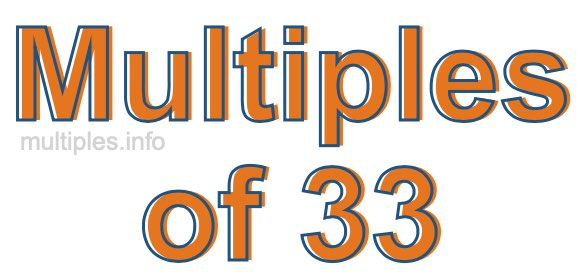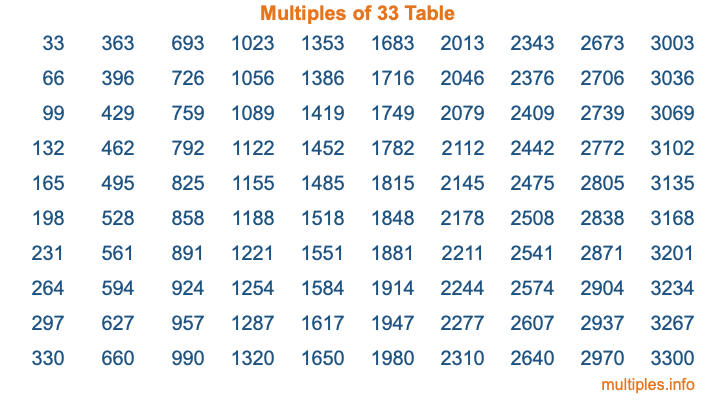Multiples of 33Welcome to the Multiples of 33 page. Here we will first teach you everything you will ever need to know about the multiples of 33, and then give you a study guide summary of everything we taught you to make sure you remember it all. Use this page to look up facts and learn information about the multiples of 33. This page will make you a multiples of thirty-three expert!

Definition of Multiples of 33
Multiples of 33 are all the numbers that when divided by 33 equal an integer. Each of the multiples of 33 are called a multiple. A multiple of 33 is created by multiplying 33 by an integer.

Therefore, to create a list of multiples of 33, you start with 1 multiplied by 33, then 2 multiplied by 33, then 3 multiplied by 33, and so on for as long as you want. Thus, the list of the first five multiples of 33 is 33, 66, 99, 132, and 165. To see a larger list of multiples of 33, see the printable image of Multiples of 33 further down on this page. We also have a category where you can choose any nth multiple of 33.

Multiples of 33 Checker
The Multiples of 33 Checker below checks to see if any number of your choice is a multiple of 33. In other words, it checks to see if there is any number (integer) that when multiplied by 33 will equal your number. To do that, we divide your number by 33. If the the quotient is an integer, then your number is a multiple of 33.

Is  a multiple of 33?

Least Common Multiple of 33 and ...
A Least Common Multiple (LCM) is the lowest multiple that two or more numbers have in common. This is also called the smallest common multiple or lowest common multiple and is useful to know when you are adding our subtracting fractions. Enter one or more numbers below (33 is already entered) to find the LCM.

Check out our LCM Calculator if you need more details about the Least Common Multiple or if you need the LCM for different numbers for adding and subtraction fractions.

nth Multiple of 33
As we stated above, 33 is the first multiple of 33, 66 is the second multiple of 33, 99 is the third multiple of 33, and so on. Enter a number below to find the nth multiple of 33.

th multiple of 33

Multiples of 33 vs Factors of 33
33 is a multiple of 33 and a factor of 33, but that is where the similarities end. All postive multiples of 33 are 33 or greater than 33. All positive factors of 33 are 33 or less than 33.

Below is the beginning list of multiples of 33 and the factors of 33 so you can compare:

Multiples of 33: 33, 66, 99, 132, 165, etc.

Factors of 33: 1, 3, 11, 33

As you can see, the multiples of 33 are all the numbers that you can divide by 33 to get a whole number. The factors of 33, on the other hand, are all the whole numbers that you can multiply by another whole number to get 33.

It's also interesting to note that if a number (x) is a factor of 33, then 33 will also be a multiple of that number (x).

Multiples of 33 vs Divisors of 33
The divisors of 33 are all the integers that 33 can be divided by evenly. Below is a list of the divisors of 33.

Divisors of 33: 1, 3, 11, 33

The interesting thing to note here is that if you take any multiple of 33 and divide it by a divisor of 33, you will see that the quotient is an integer.

Multiples of 33 Table
Below is an image of the first 100 multiples of 33 in a table. The table is in chronological order, column by column. The first column has the first ten multiples of 33, the second column has the next ten multiples of 33, and so on.The Multiples of 33 Table is also referred to as the 33 Times Table or Times Table of 33. You are welcome to print out our table for your studies.

Negative Multiples of 33
Although not often discussed or needed in math, it is worth mentioning that you can make a list of negative multiples of 33 by multiplying 33 by -1, then by -2, then by -3, and so on, to get the following list of negative multiples of 33:

-33, -66, -99, -132, -165, etc.

Multiples of 33 Summary
Below is a summary of important Multiples of 33 facts that we have discussed on this page. To retain the knowledge on this page, we recommend that you read through the summary and explain to yourself or a study partner why they hold true.

There are an infinite number of multiples of 33.

A multiple of 33 divided by 33 will equal a whole number.

33 divided by a factor of 33 equals a divisor of 33.

The nth multiple of 33 is n times 33.

The largest factor of 33 is equal to the first positive multiple of 33.

33 is a multiple of every factor of 33.

33 is a multiple of 33.

A multiple of 33 divided by a divisor of 33 equals an integer.

33 divided by a divisor of 33 equals a factor of 33.

Any integer times 33 will equal a multiple of 33.

Multiples of a Number
Here you can get the multiples of another number, all with the same attention to detail as we did for multiples of 33 on this page.

Multiples of
Multiples of 34
Did you find our page about multiples of thirty-three educational? Do you want more knowledge? Check out the multiples of the next number on our list!